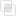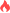python merge()的连接

Python2021-06-04 11:45:27891浏览 · 0收藏 · 0评论1、说明

pandas提供了一个类似于关系数据库的连接(join)操作的方法merage,可以根据一个或多个键将不同DataFrame中的行连接起来。

2、语法

```merge(left, right, how='inner', on=None, left_on=None, right_on=None,
left_index=False, right_index=False, sort=True,
suffixes=('_x', '_y'), copy=True, indicator=False)```

3、参数

left与right：两个不同的DataFrame

how：指的是合并(连接)的方式有inner(内连接),left(左外连接),right(右外连接),outer(全外连接);默认为inner

on : 指的是用于连接的列索引名称。必须存在右右两个DataFrame对象中，如果没有指定且其他参数也未指定则以两个DataFrame的列名交集做为连接键

left_on：左则DataFrame中用作连接键的列名;这个参数中左右列名不相同，但代表的含义相同时非常有用。

right_on：右则DataFrame中用作 连接键的列名

left_index：使用左则DataFrame中的行索引做为连接键

right_index：使用右则DataFrame中的行索引做为连接键

sort：默认为True，将合并的数据进行排序。在大多数情况下设置为False可以提高性能

suffixes：字符串值组成的元组，用于指定当左右DataFrame存在相同列名时在列名后面附加的后缀名称，默认为(’_x’,’_y’)

copy：默认为True,总是将数据复制到数据结构中；大多数情况下设置为False可以提高性能

indicator：在 0.17.0中还增加了一个显示合并数据中来源情况；如只来自己于左边(left_only)、两者(both)

4、实例

```import pandas as pd

left = pd.DataFrame({'key': ['K0', 'K1', 'K2', 'K3'],
'A': ['A0', 'A1', 'A2', 'A3'],
'B': ['B0', 'B1', 'B2', 'B3']})
right = pd.DataFrame({'key': ['K0', 'K1', 'K2', 'K3'],
'C': ['C0', 'C1', 'C2', 'C3'],
'D': ['D0', 'D1', 'D2', 'D3']})
result = pd.merge(left, right, on='key')

# on参数传递的key作为连接键
result
Out:
A   B key   C   D
0  A0  B0  K0  C0  D0
1  A1  B1  K1  C1  D1
2  A2  B2  K2  C2  D2
3  A3  B3  K3  C3  D3```python中__new__的重写python解决初始化执行次数python错误类型捕获的方法python抛出raise异常的注意点python异常的传递python模块的搜索顺序分析python__name__ 属性的使用注意Python psd-tools如何转换文件Python列表操作方法的整理Python字符串方法如何使用Python字典常用方法汇总Python中os模块的功能介绍Python curses库如何使用Python curses内置颜色怎么用Python实现Excel的整行插入Python中DataFrame有哪些常用方法Python中使用xlwings整行删除Python中concurrent.futures模块如何使用Python kmeans聚类的使用Python K-means算法的计算步骤Python默认值的使用注意Python特殊传参如何实现java虚拟机中栈的运行原理843

js中自定义迭代行为的介绍714

python中del在垃圾回收的使用852